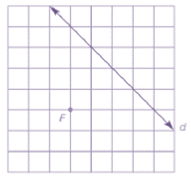Chapter 7.1, Problem 26E### Elementary Geometry for College St...

6th Edition
Daniel C. Alexander + 1 other
ISBN: 9781285195698

#### Solutions

Chapter
Section### Elementary Geometry for College St...

6th Edition
Daniel C. Alexander + 1 other
ISBN: 9781285195698
Textbook Problem
1 views

# For Exercises 21 to 26, use the grid and your compass (as needed) to locate several points on the parabola having the given focus F and directrix d . Then sketch the parabola that is characterized by these points.To determine

To sketch:

The parabola using the below given grid with directrix and focus.Explanation

Definition:

A parabola is the locus of points that are equidistant from a fixed line and a fixed point not on that line.

The fixed point is known as focus of the parabola and the fixed line is known as directrix of the parabola.

Procedure:

1. Draw the given directrix d and focus F and locate a point D1.

2. Locate a point P1 which is the mid-point of FD1. The point P1 will be the vertex.

3. Locate the points P1, P2, and P3 such that D1</

### Still sussing out bartleby?

Check out a sample textbook solution.

See a sample solution

#### The Solution to Your Study Problems

Bartleby provides explanations to thousands of textbook problems written by our experts, many with advanced degrees!

Get Started

#### Show that modus tollens is a valid form of argument.

Finite Mathematics for the Managerial, Life, and Social Sciences

#### Solve the equations in Exercises 126. 1x211+1=0

Finite Mathematics and Applied Calculus (MindTap Course List)

#### The radius of convergence of is: 1 3 ∞

Study Guide for Stewart's Single Variable Calculus: Early Transcendentals, 8th